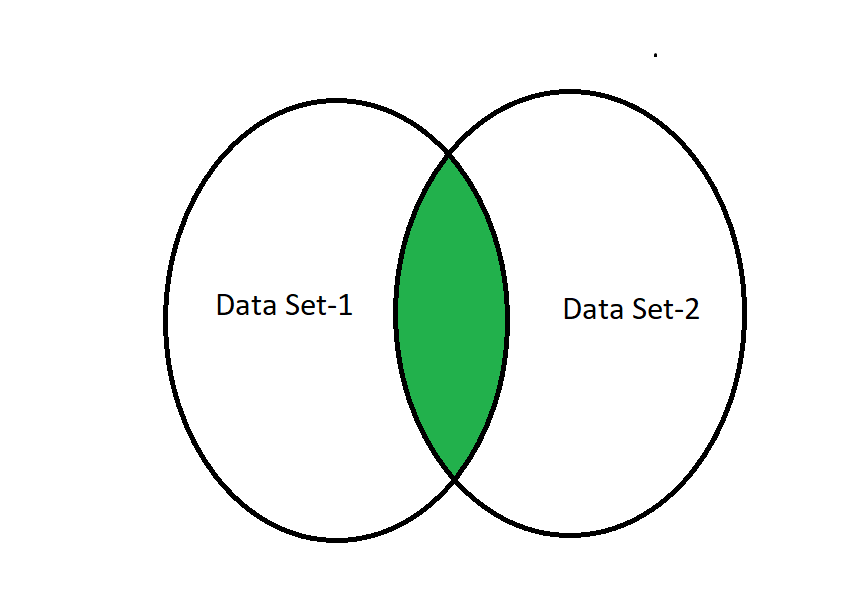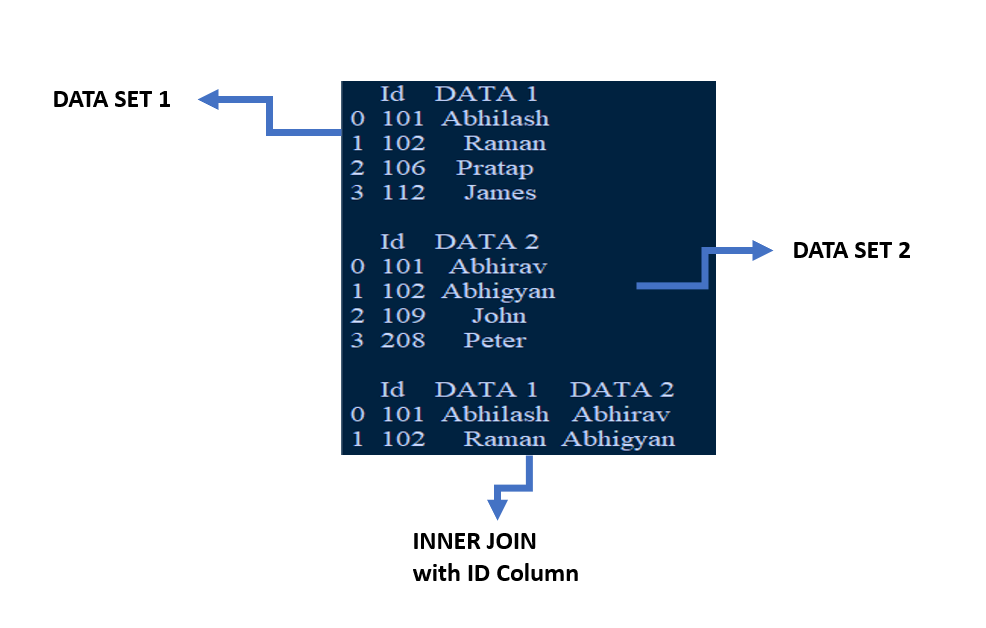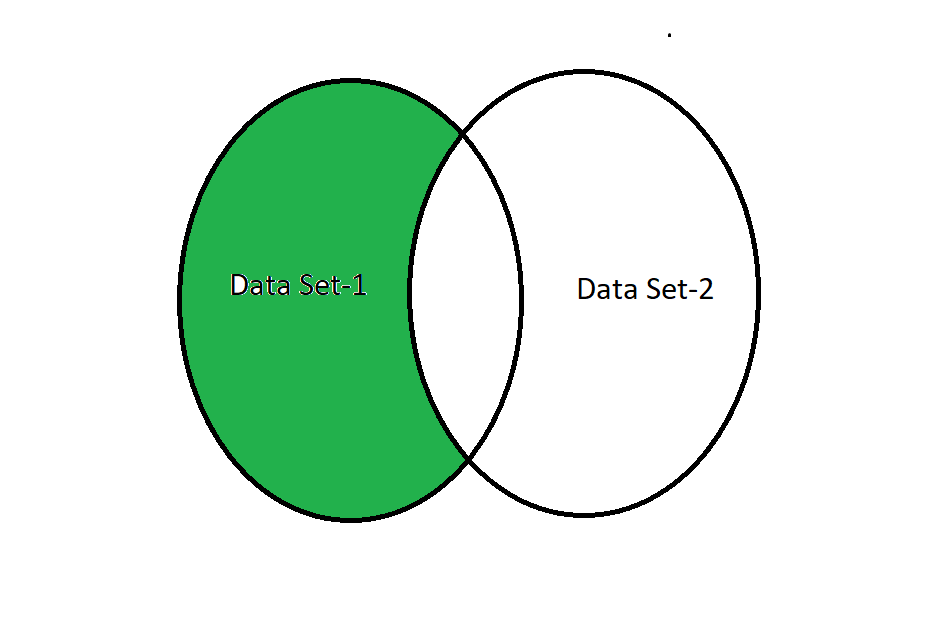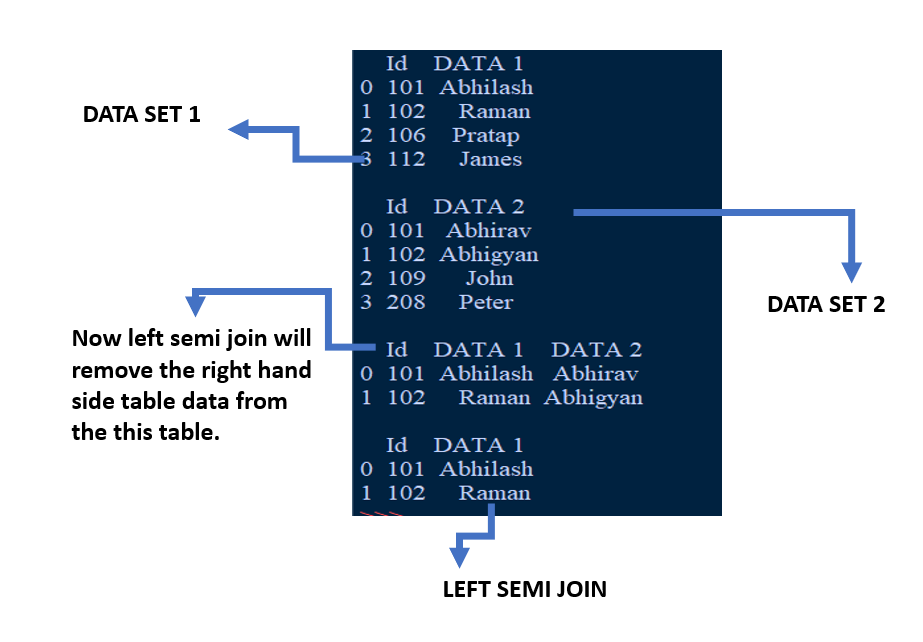Open In App

# Python Pandas – Difference between INNER JOIN and LEFT SEMI JOIN

In this article, we see the difference between INNER JOIN and LEFT SEMI JOIN.

## Inner Join

An inner join requires two data set columns to be the same to fetch the common row data values or data from the data table. In simple words, and returns a data frame or values with only those rows in the data frame that have common characteristics and behavior desired by the user. This is similar to the intersection of two sets in mathematics. In short, we can say that Inner Join on column Id will return columns from both the tables and only the matching records:Inner Join

### Example:

Suppose two companies are conducting an inter-company cricket tournament and the employees who have participated gave their names in the data set table. Now in the table, we have two or more similar Id. Now we have two sets of data tables. And we want the data of all the employees with the same ID in two different companies so that we can easily differentiate the same id in different companies. In such a scenario we will use the inner join concept to get all the details of such employees.

## Python3

 `# importing pandas as pds``import` `pandas as pds`` ` `# Creating dataframe for the data_set first``data_Set1 ``=` `pds.DataFrame()`` ` `# Creating data list for the table 1``# here Id 101 and 102 will be same like ``# in data set 2``schema ``=` `{``'Id'``: [``101``, ``102``, ``106``, ``112``],``          ``'DATA 1'``: [``'Abhilash'``, ``'Raman'``, ``'Pratap'``, ``'James'``]}``data_Set1 ``=` `pds.DataFrame(schema)`` ` `print``(``"Data Set-1 \n"``, data_Set1, ``"\n"``)`` ` `# Creating dataframe data_set second``data_Set2 ``=` `pds.DataFrame()`` ` `# Creating data list for the table 2``# here Id 101 and 102 will be same like ``# in data set 1``schema ``=` `{``'Id'``: [``101``, ``102``, ``109``, ``208``],``          ``'DATA 2'``: [``'Abhirav'``, ``'Abhigyan'``, ``'John'``, ``'Peter'``]}``data_Set2 ``=` `pds.DataFrame(schema)`` ` `print``(``"Data Set-2 \n"``, data_Set2, ``"\n"``)`` ` `# inner join in python``inner_join ``=` `pds.merge(data_Set1, data_Set2, on``=``'Id'``, how``=``'inner'``)`` ` `# display dataframe``pds.DataFrame(inner_join)`

Output:## Left Semi-Join

A left semi-join requires two data set columns to be the same to fetch the data and returns all columns data or values from the left dataset, and ignores all column data values from the right dataset. In simple words, we can say that Left Semi Join on column Id will return columns only from the left table and matching records only from the left table.Left Semi-Join

### Example:

Suppose two companies are conducting an inter-company Cricket tournament and the employees who have participated gave their names in the data set table. Now in the table, we have two or more similar Id. Now we have two sets of data tables. Companies with the data on the left-hand side want to give priority to the employee of their company so that they can choose who will play first.

## Python3

 `# importing pandas as pds``import` `pandas as pds`` ` `# Creating dataframe for the data_set first``data_Set1 ``=` `pds.DataFrame()`` ` `# Creating data list for the table 1``schema ``=` `{``'Id'``: [``101``, ``102``, ``106``, ``112``],``     ``'DATA 1'``: [``'Abhilash'``, ``'Raman'``, ``'Pratap'``, ``'James'``]} ``data_Set1``=` `pds.DataFrame(schema)``print``(data_Set1,``"\n"``)`` ` `# Creating dataframe data_set second``data_Set2 ``=` `pds.DataFrame()`` ` `# Creating data list for the table 2``schema2 ``=` `{``'Id'``: [``101``, ``102``, ``109``, ``208``],``     ``'DATA 2'``: [``'Abhirav'``, ``'Abhigyan'``, ``'John'``, ``'Peter'``]}``data_Set2``=` `pds.DataFrame(schema2)`` ` `print``(data_Set2,``"\n"``) `` ` `# setting the base for the left semi-join in python``semi``=``data_Set1.merge(data_Set2,on``=``'Id'``)``print``(semi,``"\n"``)``data_Set1[``'Id'``].isin(data_Set2[``'Id'``])``semi``=``data_Set1.merge(data_Set2,on``=``'Id'``)`` ` `# our left semi join``new_semi``=``data_Set1[data_Set1[``'Id'``].isin(semi[``'Id'``])]``pds.DataFrame(new_semi)`

Output: